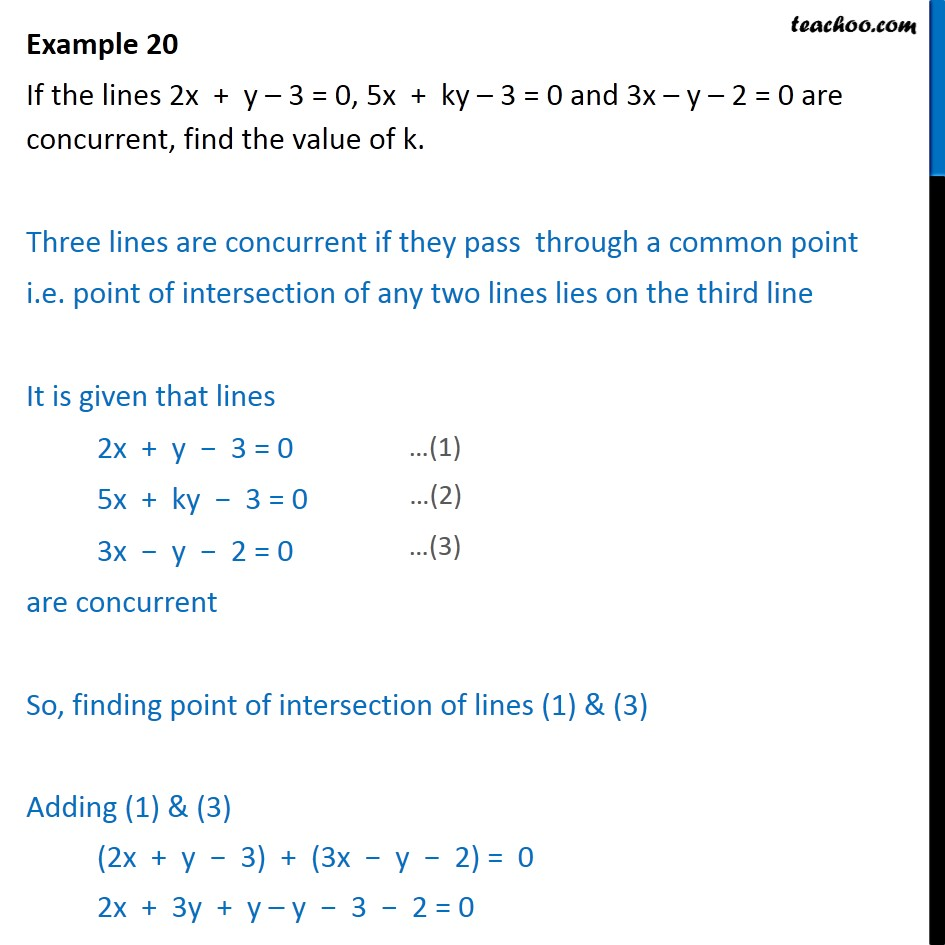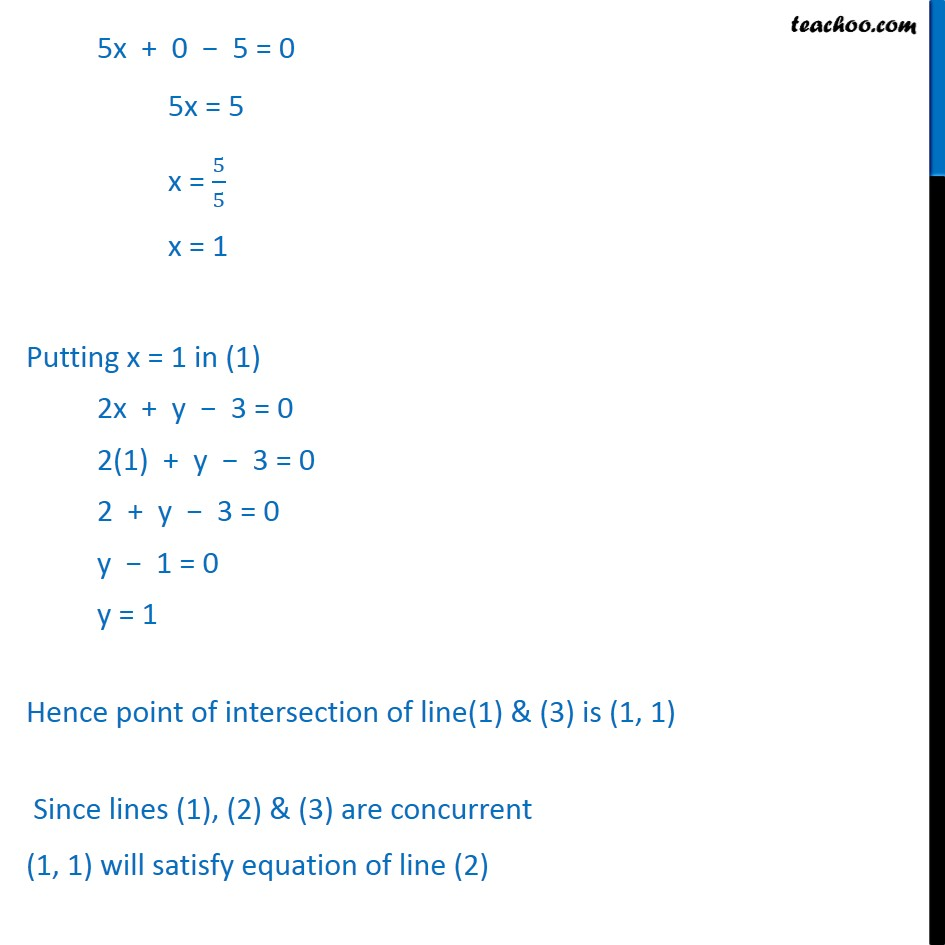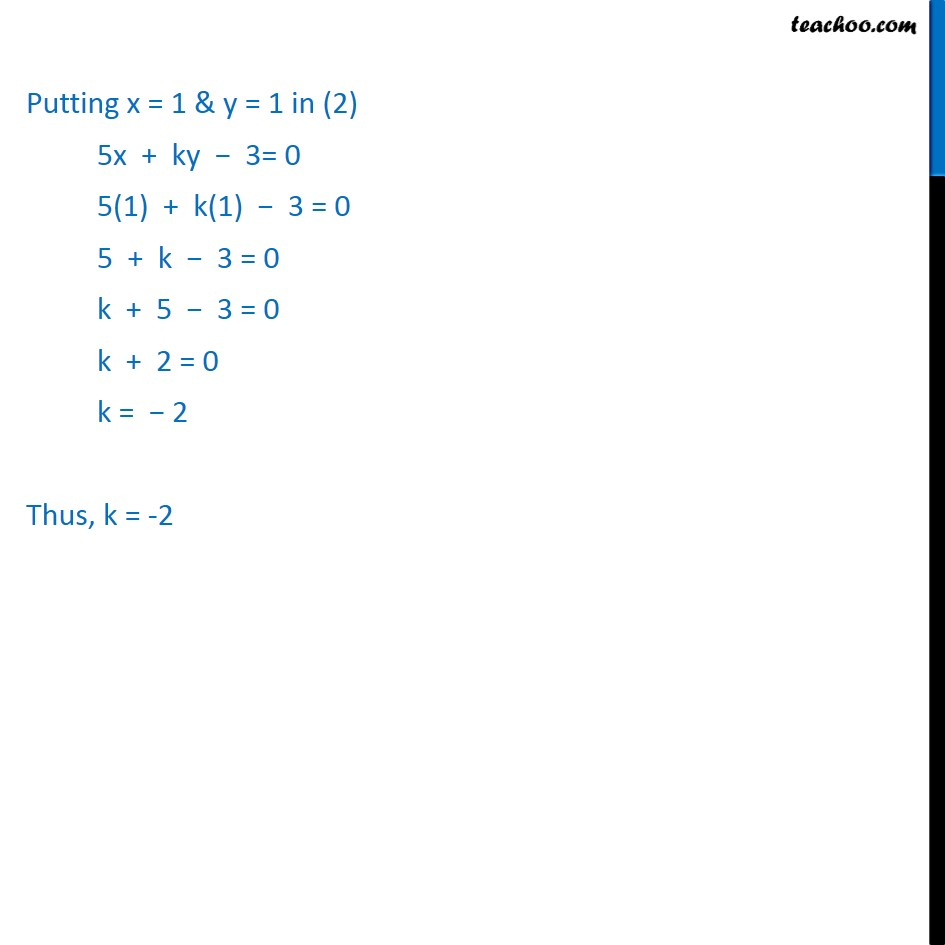1. Chapter 10 Class 11 Straight Lines
2. Concept wise
3. Other Type of questions - 3 lines Concurrent

Transcript

Example 20 If the lines 2x + y 3 = 0, 5x + ky 3 = 0 and 3x y 2 = 0 are concurrent, find the value of k. Three lines are concurrent if they pass through a common point i.e. point of intersection of any two lines lies on the third line It is given that lines 2x + y 3 = 0 5x + ky 3 = 0 3x y 2 = 0 are concurrent So, finding point of intersection of lines (1) & (3) Adding (1) & (3) (2x + y 3) + (3x y 2) = 0 2x + 3y + y y 3 2 = 0 5x + 0 5 = 0 5x = 5 x = 5/5 x = 1 Putting x = 1 in (1) 2x + y 3 = 0 2(1) + y 3 = 0 2 + y 3 = 0 y 1 = 0 y = 1 Hence point of intersection of line(1) & (3) is (1, 1) Since lines (1), (2) & (3) are concurrent (1, 1) will satisfy equation of line (2) Putting x = 1 & y = 1 in (2) 5x + ky 3= 0 5(1) + k(1) 3 = 0 5 + k 3 = 0 k + 5 3 = 0 k + 2 = 0 k = 2 Thus, k = -2

Other Type of questions - 3 lines Concurrent